CAT  >  Test: Logarithm- 3

# Test: Logarithm- 3

Test Description

## 15 Questions MCQ Test Quantitative Aptitude (Quant) | Test: Logarithm- 3

Test: Logarithm- 3 for CAT 2023 is part of Quantitative Aptitude (Quant) preparation. The Test: Logarithm- 3 questions and answers have been prepared according to the CAT exam syllabus.The Test: Logarithm- 3 MCQs are made for CAT 2023 Exam. Find important definitions, questions, notes, meanings, examples, exercises, MCQs and online tests for Test: Logarithm- 3 below.
Solutions of Test: Logarithm- 3 questions in English are available as part of our Quantitative Aptitude (Quant) for CAT & Test: Logarithm- 3 solutions in Hindi for Quantitative Aptitude (Quant) course. Download more important topics, notes, lectures and mock test series for CAT Exam by signing up for free. Attempt Test: Logarithm- 3 | 15 questions in 15 minutes | Mock test for CAT preparation | Free important questions MCQ to study Quantitative Aptitude (Quant) for CAT Exam | Download free PDF with solutions
 1 Crore+ students have signed up on EduRev. Have you?
Test: Logarithm- 3 - Question 1

### If 3X+I = 6log23 then x is

Detailed Solution for Test: Logarithm- 3 - Question 1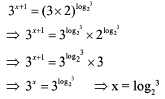Test: Logarithm- 3 - Question 2

### log5P x logp x = 2. What is the value of x?

Detailed Solution for Test: Logarithm- 3 - Question 2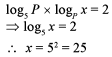Test: Logarithm- 3 - Question 3

### If [N] = the greatest integer less than or equal to N, then [log10 6730.4] is equal to

Detailed Solution for Test: Logarithm- 3 - Question 3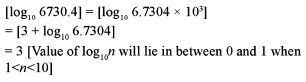Test: Logarithm- 3 - Question 4

What is the number of zeros coming immediately after the decimal point in the value of (0.2)25 ? (log102 = 0.30103)

Detailed Solution for Test: Logarithm- 3 - Question 4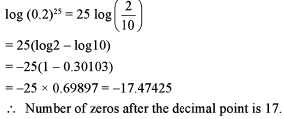Test: Logarithm- 3 - Question 5

Find the value of log64(l/16).

Detailed Solution for Test: Logarithm- 3 - Question 5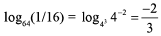Test: Logarithm- 3 - Question 6

Arrange the following in an ascending order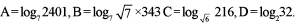Detailed Solution for Test: Logarithm- 3 - Question 6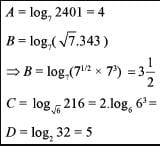A = 4, B = 7/2, C = 6, D = 5

Test: Logarithm- 3 - Question 7

What is the value of x if log3x + log9x + log27x + log81x  = 25/4?

Detailed Solution for Test: Logarithm- 3 - Question 7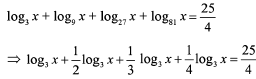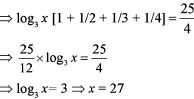Test: Logarithm- 3 - Question 8

What is the value of x in the following expression: log2 ( 3 - x ) + log2 ( 1 - x ) = 3?

Detailed Solution for Test: Logarithm- 3 - Question 8

Going through options.
Option (a) is not possible.
Checking option (c): Forx = -1, expression = log24 + log22 = log28 = 31og22 = 3

Test: Logarithm- 3 - Question 9

What is the value of log3√3 27 ?

Detailed Solution for Test: Logarithm- 3 - Question 9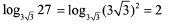Test: Logarithm- 3 - Question 10

What is the value of P if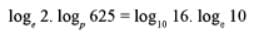?

Detailed Solution for Test: Logarithm- 3 - Question 10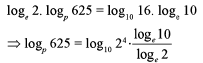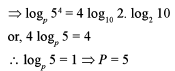Test: Logarithm- 3 - Question 11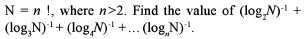Detailed Solution for Test: Logarithm- 3 - Question 11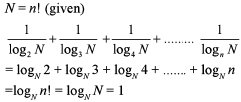Test: Logarithm- 3 - Question 12

If x = loga (bc), y = logb (ca) and z = logc (ab) when which of the following is equal to 1?

Detailed Solution for Test: Logarithm- 3 - Question 12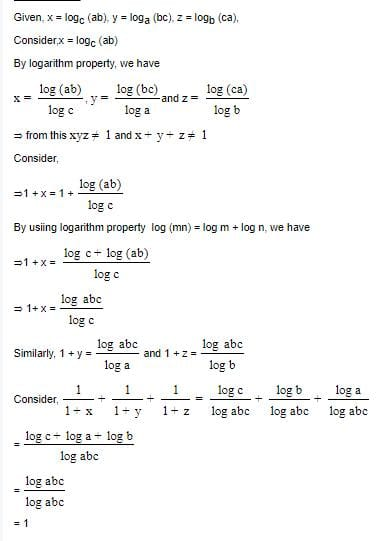Test: Logarithm- 3 - Question 13

If logk x.log5 k = 1 k ≠ 1.k > 0, then x is equal to

Detailed Solution for Test: Logarithm- 3 - Question 13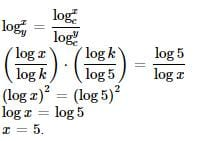Test: Logarithm- 3 - Question 14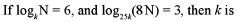Detailed Solution for Test: Logarithm- 3 - Question 14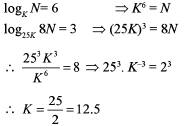Test: Logarithm- 3 - Question 15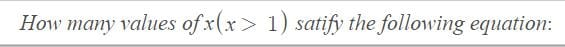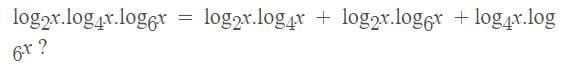Detailed Solution for Test: Logarithm- 3 - Question 15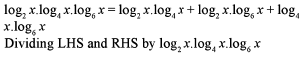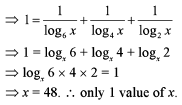## Quantitative Aptitude (Quant)

163 videos|163 docs|131 tests
 Use Code STAYHOME200 and get INR 200 additional OFF Use Coupon Code
Information about Test: Logarithm- 3 Page
In this test you can find the Exam questions for Test: Logarithm- 3 solved & explained in the simplest way possible. Besides giving Questions and answers for Test: Logarithm- 3, EduRev gives you an ample number of Online tests for practice

## Quantitative Aptitude (Quant)

163 videos|163 docs|131 tests

### How to Prepare for CAT

Read our guide to prepare for CAT which is created by Toppers & the best Teachers